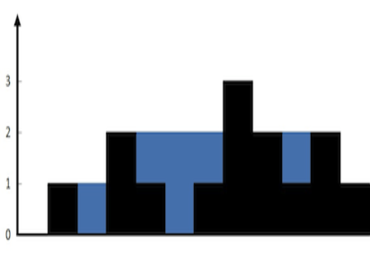Category

# Interview Questions

Too many people stumble through job interviews as if the questions asked are coming out of left field. But many job interview questions are to be expected.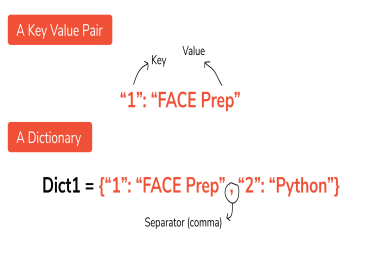## Write a function to flatten a nested dictionary separated by period

Write a function to flatten a nested dictionary separated by period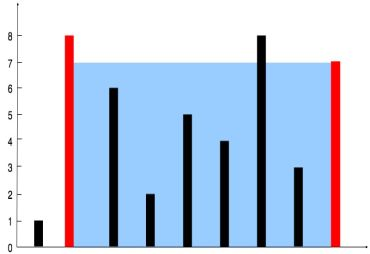## Problem: Container With Most Water

Given n non-negative integers a1, a2, …, an , where each represents a point at coordinate (i, ai). n vertical lines are drawn such that the two endpoints of line i is at (i, ai) and (i, 0)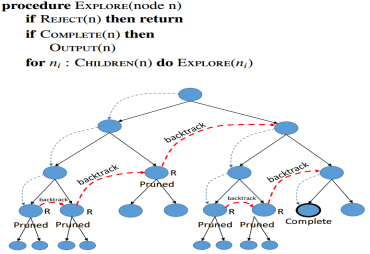## An Introduction To Backtracking

Backtracking reduces the search space since we no longer have to follow down any paths we know are invalid. This is called...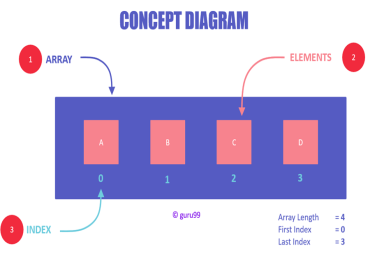## A maximal subarray

find the contiguous subarray of arr with the maximal sum of items...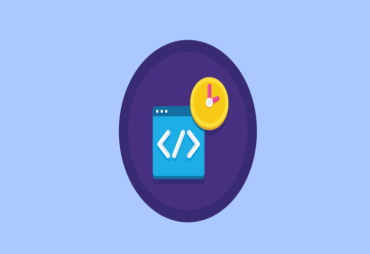## Write a function that prints the least integer that is not present in a given list and cannot be represented by the summation of the sub-elements of the list

Write a function that prints the least integer that is not present in a given list and cannot be represented by the summation of the sub-elements of the list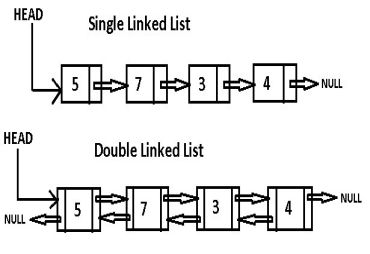## Rearrange linked list into an alternating high - low values

Given a linked list, rearrange the node values such that they appear in alternating low -> high -> low -> high ... form.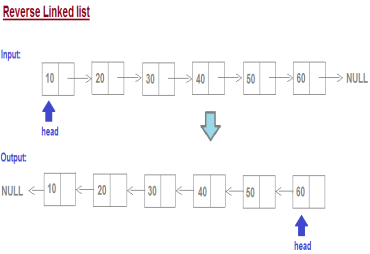## Reverse a linked list using recursion and without recursion

Reverse a linked list using recursion and without recursion. A linked list is a linear data structure where each element is a separate object. Linked list elements are not stored at the contiguous location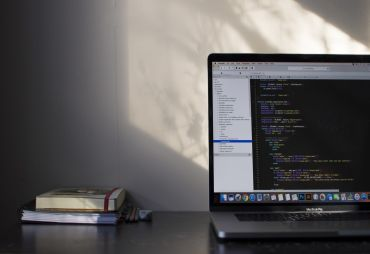## Given an array of integers, return a new array where each element in the new array is the number of smaller elements to the right of that element in the original input array.

Given an array of integers, return a new array where each element in the new array is the number of smaller elements to the right of that element in the original input array.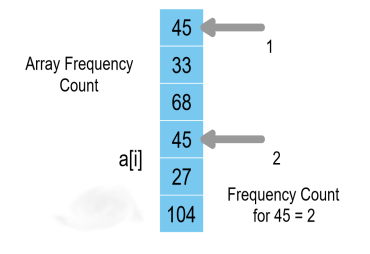## Frequency of each character in string

The problem is to print the characters along with their frequency and in the given format explained in the examples below